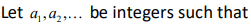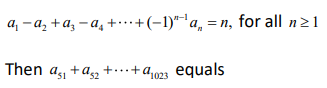# Let a(base1), a(base2) | Quantitative Aptitude – Sequence and series

## CAT 2019 – Slot -2 - Question 4 - Let a(base1), a(base2)Let a(base1), a(base2)1. 1
2. −1
3. 0
4. 10

Given , a(base1) – a(base2) + a(base3) – a(base4)+ ……+ (-1)^(n-1) a(base n) = n

Put n = 1, a(base1) = 1

Put n =2 , a(base1) – a(base2) = 2 or 1 – a(base2) = 2

Thus a(base2) = -1

Put n =3, a(base1) – a(base2) + a(base3) = 3, by solving a(base3) = 1

Similarly you will get a(base4) = -1, a(base5) = 1 ..and so on

Thus we can say all odd numbered = 1 all even numbered = -1

We are now asked to find out

a(base51) + a(base52) + …+ a(base1023) = 1 + (-1) + 1 + (-1)…

Starting with a(51), if the total number of terms is even: all terms will cancel out and the result will be 0.

So,

a(51) + a(52) = 0

a(51) + a(52) + a(53) + a(54) = 0

a(51) + a(52) + a(53) + a(54) … a(1022)= 0

a(51) + a(52) + a(53) + a(54) … a(1022) + a(1023)= 0 + a(1023) = 0 + 1 = 1

So, our answer for this sum would be 1.

### Past Year Question Paper & SolutionsCounselling Session
By IIM Mentor

#### Free Material Area